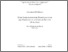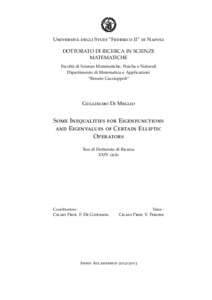Ricerca
Browse
Informazioni

Di Meglio, Guglielmo (2013) Some Inequalities for Eigenfunctions and Eigenvalues of Certain Elliptic Operators. [Tesi di dottorato]Preview
Text
Tesi_Di_Meglio-def.pdf

[error in script] [error in script]
Item Type: Tesi di dottorato
Lingua: English
Title: Some Inequalities for Eigenfunctions and Eigenvalues of Certain Elliptic Operators
Creators:
CreatorsEmail
Di Meglio, Guglielmoguglielmo.dimeglio@unina.it
Date: 2 April 2013
Institution: Università degli Studi di Napoli Federico II
Department: Matematica e applicazioni "Renato Caccioppoli"
Scuola di dottorato: Scienze matematiche e informatiche
Dottorato: Scienze matematiche
Ciclo di dottorato: 24
Coordinatore del Corso di dottorato:
nomeemail
De Giovanni, Francescodegiovanni@unina.it
Tutor:
nomeemail
Ferone, Vincenzoferone@unina.it
Date: 2 April 2013
Uncontrolled Keywords: Rearrangements, symmetrized eigenfunctions, principal eigenvalue, Faber-Krahn inequality
Settori scientifico-disciplinari del MIUR: Area 01 - Scienze matematiche e informatiche > MAT/05 - Analisi matematica
Date Deposited: 14 Apr 2013 14:31We present some geometric and analytic inequalities related to solutions of certain elliptic PDEs. In chapter 1, we introduced the symmetrization techniques which will be used through the paper. In chapter 2, we prove a family of isoperimetric inequalities for bodies of revolution which arise in connection with the problem of finding the extremals in some Hardy-Sobolev inequality. In particular, we are able to prove that the inequalities are sharp and that a characterization of the equality case is available, yielding the best constant. In chapter 3, we prove two stability type estimates which involve the symmetrized normalized first eigenfunction of certain elliptic operators (modelled on the Laplacian). In particular, we prove that the $L^\infty$-distance of the symmetrized first eigenfunction from the first eigenfunction of a suitable symmetrized problem can be controlled using the distance between the first eigenvalues of such problems. In chapter 4, we prove a generalization of the classical Faber-Krahn inequality for the principal weighted eigenvalue of $p$-Laplace operator plus an indefinite potential.